CAT  >  Maths and Logical Reasoning Test- 1

# Maths and Logical Reasoning Test- 1

Test Description

## 15 Questions MCQ Test TISS-NET Section Wise & Full Mock Test Series | Maths and Logical Reasoning Test- 1

Maths and Logical Reasoning Test- 1 for CAT 2022 is part of TISS-NET Section Wise & Full Mock Test Series preparation. The Maths and Logical Reasoning Test- 1 questions and answers have been prepared according to the CAT exam syllabus.The Maths and Logical Reasoning Test- 1 MCQs are made for CAT 2022 Exam. Find important definitions, questions, notes, meanings, examples, exercises, MCQs and online tests for Maths and Logical Reasoning Test- 1 below.
Solutions of Maths and Logical Reasoning Test- 1 questions in English are available as part of our TISS-NET Section Wise & Full Mock Test Series for CAT & Maths and Logical Reasoning Test- 1 solutions in Hindi for TISS-NET Section Wise & Full Mock Test Series course. Download more important topics, notes, lectures and mock test series for CAT Exam by signing up for free. Attempt Maths and Logical Reasoning Test- 1 | 15 questions in 15 minutes | Mock test for CAT preparation | Free important questions MCQ to study TISS-NET Section Wise & Full Mock Test Series for CAT Exam | Download free PDF with solutions
 1 Crore+ students have signed up on EduRev. Have you?
Maths and Logical Reasoning Test- 1 - Question 1

### Four number-pairs have been given, out of which three are alike in some manner and one is different. Choose out the odd one.

Detailed Solution for Maths and Logical Reasoning Test- 1 - Question 1

The pattern followed here is,

Option (A) → 9 : 738 → 738 = 93 + 9

Option (B) → 6 : 210 → 210 = 63 - 6

Option (C) → 8 : 504 → 504 = 83 - 8

Option (D) → 7 : 336 → 336 = 73 - 7

All option follow the same pattern, except ‘9 : 738’

Hence, the correct option is (A).

Maths and Logical Reasoning Test- 1 - Question 2

### Select the missing number from the given series?3, 11, 38, 102, ?

Detailed Solution for Maths and Logical Reasoning Test- 1 - Question 2

The pattern followed is,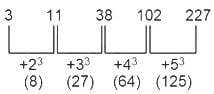Hence, the correct option is (C).

Maths and Logical Reasoning Test- 1 - Question 3

### Direction: In the following question below are given some statements followed by some conclusions. Taking the given statements to be true even if they seem to be at variance from commonly known facts, read all the conclusions and then decide which of the given conclusions logically follows the given statements.Statements:I. All doors are room.II. No room is a window.Conclusions:I. No door is a window.II. No window is a room.

Detailed Solution for Maths and Logical Reasoning Test- 1 - Question 3

The least possible Venn Diagram for the given statements will be as follows,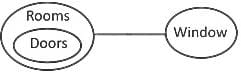I. No door is a window → True (As shown in the figure).

II. No window is a room → As no room is a window. Thus, no window is a room. So, true.

Hence, the correct option is (C).

Maths and Logical Reasoning Test- 1 - Question 4

Identify the odd one from the given groups of letters.

Detailed Solution for Maths and Logical Reasoning Test- 1 - Question 4

In all options 1st letter is vowel and second is opposite of that vowel except CX.

Hence, the correct option is (D).

Maths and Logical Reasoning Test- 1 - Question 5

Direction: Answer the following question based on the information given below.

The table below shows the number of cases of various crimes reported at police stations in different states in 2015-16.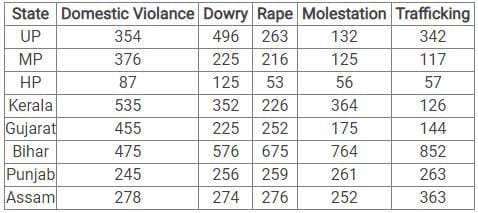What is the ratio of molestation cases and rape cases?

Detailed Solution for Maths and Logical Reasoning Test- 1 - Question 5

Total cases reported on Molestation = 132 + 125 + 56 + 364 + 175 + 764 + 261 + 252 = 2129

Total cases reported on Rape = 263 + 216 + 53 + 226 + 252 + 675 + 259 + 276 = 2220

∴ Required ratio = 2129 : 2220 = 0.96

Hence, the correct option is (B).

Maths and Logical Reasoning Test- 1 - Question 6

Direction: Answer the following question based on the information given below.

The table below shows the number of cases of various crimes reported at police stations in different states in 2015-16.

In which state are the least number of cases reported?

Detailed Solution for Maths and Logical Reasoning Test- 1 - Question 6

This question can be solved very quickly by observation.

HP has the lowest reported cases for each crime. Thus, it will have the least number of cases across all crimes.

Hence, the correct option is (D).

Maths and Logical Reasoning Test- 1 - Question 7

Direction: Answer the following question based on the information given below.

The table below shows the number of cases of various crimes reported at police stations in different states in 2015-16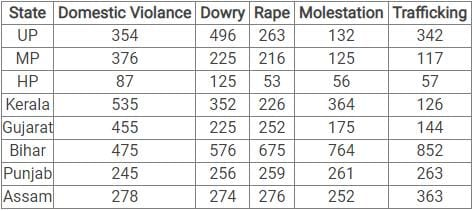The Domestic violance and Dowry cases reported in Bihar are what percent more/less than the Rape and Trafficking cases reported in Punjab?

Detailed Solution for Maths and Logical Reasoning Test- 1 - Question 7

From the table, Domestic Violance and Dowry in Bihar = 475 + 576 = 1051

Rape and Trafficking in Punjab = 259 + 263 = 522

Difference = 1051 − 522 = 529

∴ Required percentage = 529/522 x 100 = 101.3 % more

Hence, the correct option is (B).

Maths and Logical Reasoning Test- 1 - Question 8

Direction: Answer the following question based on the information given below.

The table below shows the number of cases of various crimes reported at police stations in different states in 2015-16.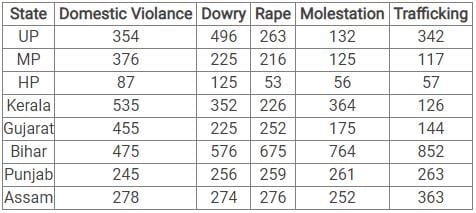The total number of Dowry and Molestation cases reported in UP is approximate what percent of the total cases reported in that state, across all crimes?

Detailed Solution for Maths and Logical Reasoning Test- 1 - Question 8

Total number of Dowry and Molestation cases reported in UP = 496 + 132 = 628

Total cases reported in UP = 628 + 354 + 263 + 342 = 1587

The closest value in the options is 40%.

∴ Required percentage = 628/1587 x 100 = 101.3 % more

Hence, the correct option is (A).

Maths and Logical Reasoning Test- 1 - Question 9

City P is to the East of city Q and city R is to the south of city P. If city S is to the west of city R, then in which direction is city S located in respect of city P?

Detailed Solution for Maths and Logical Reasoning Test- 1 - Question 9

Drawing the diagram according to the given information,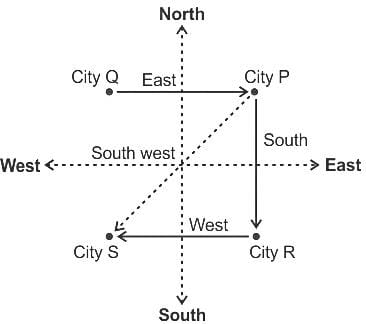So, city S is located in South West direction with respect to city P.

Hence, the correct option is (C).

Maths and Logical Reasoning Test- 1 - Question 10

A train 220 m long is running at a speed of 36 km /hr. What time will it take to cross a 110m long tunnel?

Detailed Solution for Maths and Logical Reasoning Test- 1 - Question 10

Given:

Length of the train =220 m

Length of the tunnel =110 m

Length of the train + Length of the tunnel = (220+110) m = 330 m

Speed of the train = 36 km/h

Speed of the train = 36 x (5/18)m/s = 10 m/sec

∴ Time taken is 33 seconds.

Hence, the correct option is (D).

Maths and Logical Reasoning Test- 1 - Question 11

In the following questions, a series is given with one term missing. Choose the correct alternative from the given options.

7, 20, 59, 176, ____ , 1580

Detailed Solution for Maths and Logical Reasoning Test- 1 - Question 11

The pattern followed here is as below:-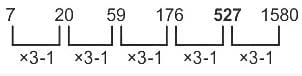Hence, the correct option is (A).

Maths and Logical Reasoning Test- 1 - Question 12

Six People A, B, C, D, E and F, are sitting in two rows with three people in each row. E is not sitting at any end of any row. D is sitting on the left of F and is at second place. C is sitting next to E and diagonally opposite to D. B is sitting next to F. On the basis of given information who is sitting opposite to B?

Detailed Solution for Maths and Logical Reasoning Test- 1 - Question 12

People - A, B, C, D, E and F, are sitting in two rows with three people in each row.

1. E is not sitting at any end of any row.

2. D is sitting on the left of F and is at second place.

3. C is sitting next to E and diagonally opposite to D.

4. B is sitting next to F.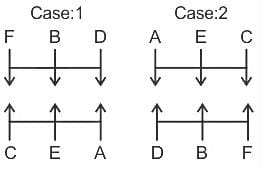Clearly, E is sitting opposite to B.

Hence, the correct option is (D).

Maths and Logical Reasoning Test- 1 - Question 13

A and B together can do a piece of work in 12 days while A alone can do the same work in 30 days. B alone can do it in

Detailed Solution for Maths and Logical Reasoning Test- 1 - Question 13

A and B together can do a piece of work in 12 days.

(A+B)’ s 1 day's work = 1/12

A alone can do the same work in 30 days.

A's 1 day's work = 1/30

So, B's 1 day's work = (1/12) - (1/30) = 1/20

∴ B alone can do the same work in 20 days.

Hence, the correct option is (D).

Maths and Logical Reasoning Test- 1 - Question 14

Find the surface area of a sphere of radius 14 cm.

Detailed Solution for Maths and Logical Reasoning Test- 1 - Question 14

Given:

Total surface area of the sphere = 4πR2,

Here R is radius.

⇒ Surface area of sphere = 4 x (22/7) x 14 x 14

⇒ Surface area of sphere =2464 cm2

Hence, the correct option is (C).

Maths and Logical Reasoning Test- 1 - Question 15

The score of 9 Students are : 7,5,4,3,9,8,2,6,5. Then find the median of the score

Detailed Solution for Maths and Logical Reasoning Test- 1 - Question 15

Given:

The score of the students 7, 5, 4, 3, 9, 8, 2, 6, 5

If N is odd, then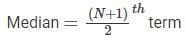Arrange the score in ascending order,

2,3,4,5,6,7,8,9

Total number = 9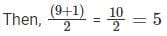Hence, the correct option is (D).

## TISS-NET Section Wise & Full Mock Test Series

28 tests
 Use Code STAYHOME200 and get INR 200 additional OFF Use Coupon Code
Information about Maths and Logical Reasoning Test- 1 Page
In this test you can find the Exam questions for Maths and Logical Reasoning Test- 1 solved & explained in the simplest way possible. Besides giving Questions and answers for Maths and Logical Reasoning Test- 1, EduRev gives you an ample number of Online tests for practice

## TISS-NET Section Wise & Full Mock Test Series

28 tests

### How to Prepare for CAT

Read our guide to prepare for CAT which is created by Toppers & the best Teachers